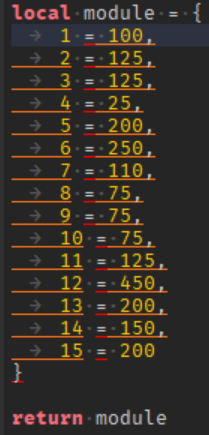# Calling module from script breaks other script

Hi, I recently added a module script (my first one ever ) but, I have one problem. On another script I have, it is basically a safezone script and when i added “tostring(data[stagetrans.Text])” , it just broke and gives me an error on another script: Main:20: attempt to perform arithmetic (sub) on nil and number. If you could figure out this problem because I tried and did nothing it would be greatly appreciated :)) Here is my current code:

``````-- // Safe zone code \\

local SoundRegionsWorkspace = workspace.SafeZones
local plr = game.Players.LocalPlayer
local steps = plr.PlayerGui.StepsGUI.Frame.TextLabel
local stagetrans = plr.PlayerGui.StageTransfer.CurrentStage
local mod = script.Parent:WaitForChild('StoreSteps')
local data = require(mod)

function whileloop()
local Found = false
for i, v in pairs(SoundRegionsWorkspace:GetChildren()) do
local region = Region3.new(v.Position - (v.Size/2),v.Position + (v.Size/2))
local parts = game.Workspace:FindPartsInRegion3WithWhiteList(region, plr.Character:GetDescendants())
for _, part in pairs(parts) do
-- Loop one by one through the parts table
if part:FindFirstAncestor(game.Players.LocalPlayer.Name) then
Found = true
break
end
end
if Found == true then
break
end
end
steps.Text = if Found or "nil" then "inf" else tostring(data[stagetrans.Text])
break
end
end

for _,v in pairs(SoundRegionsWorkspace:GetChildren()) do
v.Touched:Connect(function()
whileloop()
end)
end

-- // Main \\

local player = game.Players.LocalPlayer
local character = player.Character
local Humanoid = player.Character:WaitForChild("Humanoid")
local steps = player.PlayerGui.StepsGUI.Frame.TextLabel
local stagetrans = player.PlayerGui.StageTransfer.CurrentStage
local mod = script.Parent.StoreSteps
local RunService = game:GetService("RunService")
print(steps.Text)

local data = require(mod)

stagetrans:GetPropertyChangedSignal("Text"):Connect(function()
steps.Text = tostring(data[stagetrans.Text])
end)

print('loop')
if Humanoid.MoveDirection ~= Vector3.new(0, 0, 0) then
local new = tonumber(steps.Text)-1 -- Error on this line
local text = tostring(new)
player.PlayerGui.StepsGUI.Frame.TextLabel.Text = text
if new <= 15 then
steps.TextColor3 = Color3.new(0.866667, 0, 0.0156863)
steps.Parent.ImageLabel.ImageColor3 = Color3.new(0.866667, 0, 0.0156863)
elseif new >= 16 then
steps.TextColor3 = Color3.new(255,255,255)
steps.Parent.ImageLabel.ImageColor3 = Color3.new(255,255,255)
end
if new <= 0 then
Humanoid.Health -=100
steps.Text = tostring(data[stagetrans.Text])
break
end
end
end
``````

Where is this?

3030303030303030303030

If you scroll down I broke it up into 2 scripts; its under main.

``````local new = tonumber(steps.Text)

print(new -= 1) -- Example

``````

How would this fix the problem though? Because I am doing it like that and I want it to transfer across the whole script like a countdown not in print()

Try this:

``````local new = tonumber(steps.Text)
local NewValue = new - 1
steps.Text = NewValue
``````

I tried that code but it still gives the same error as before.

What is `steps.Text`? Is it a number?

Its a string.

iowehfosdjolfsdffsdsdf

Yes, but what type of string is it?

What do you mean?
qwdlkjnedlkd

I mean like what does it say???

It says the amount of footsteps the player has.

Ok, so can you please show the module script? That may be the issue

``````local module = {
["1"] = 100,
["2"] = 125,
["3"] = 125,
["4"] = 25,
["5"] = 200,
["6"] = 250,
["7"] = 110,
["8"] = 75,
["9"] = 75,
["10"] = 75,
["11"] = 125,
["12"] = 450,
["13"] = 200,
["14"] = 150,
["15"] = 200
}

return module
``````

Im not sure but i think its supposed to be;

`````` -- This
["1"] -- Not this

``````

That way, you dont need to keep doing `tonumber`
(Im currently on mobile, sorry for my spelling)

Everything is errorOh wait nevermind I misread SORRY

in your while loop right before the break remove the if from the assignment of steps.Text

``````steps.Text = -->if<-- Found or "nil" then "inf" else tostring(data[stagetrans.Text])
``````

Still the same error as the previous ones.